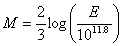Richter Scale

The Richter scale is the best known scale for measuring the magnitude of earthquakes. The magnitude value is proportional to the logarithm of the amplitude of the strongest wave during an earthquake. A recording of 7, for example, indicates a disturbance with ground motion 10 times as large as a recording of 6. The energy released by an earthquake increases by a factor of 30 for every unit increase in the Richter scale.

When working with Richter scale problems, use the following formula:where M is the magnitude and E is the amount of energy released in ergs.